### Home > MC1 > Chapter 6 > Lesson 6.3.2 > Problem6-132

6-132.
1. . Simplify each of the following expressions. Homework Help ✎

1. (4 + 8) ÷ 12 + 23

2. 3 · (8 − 5) + 6 + 2 · 7

3. 49 ÷ 7 · 5 + 4 · (3 + 2)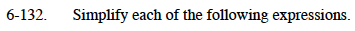For parts (a), (b), and (c), refer to the Math Notes box below. The Order of Operations is very important when simplifying expressions.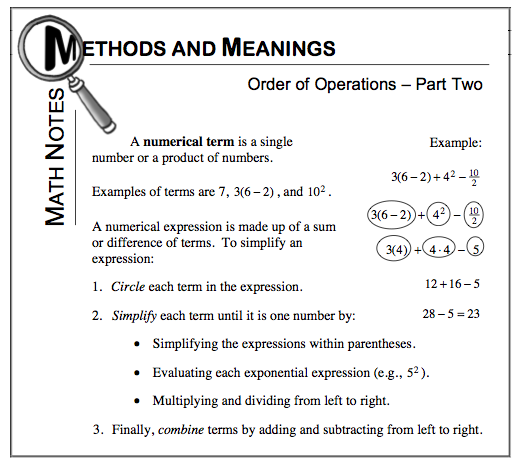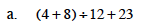24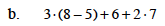Be sure to simplfy the expression inside of the parentheses before multiplying from left to right.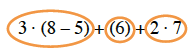Make sure you carry out each multiplication operation before using addition! The terms are circled above.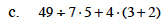Have you circled the terms?

Make sure you read the Math Notes closely! It is important to multiply and divide from left to right, or you may simplify the expression incorrectly.

55